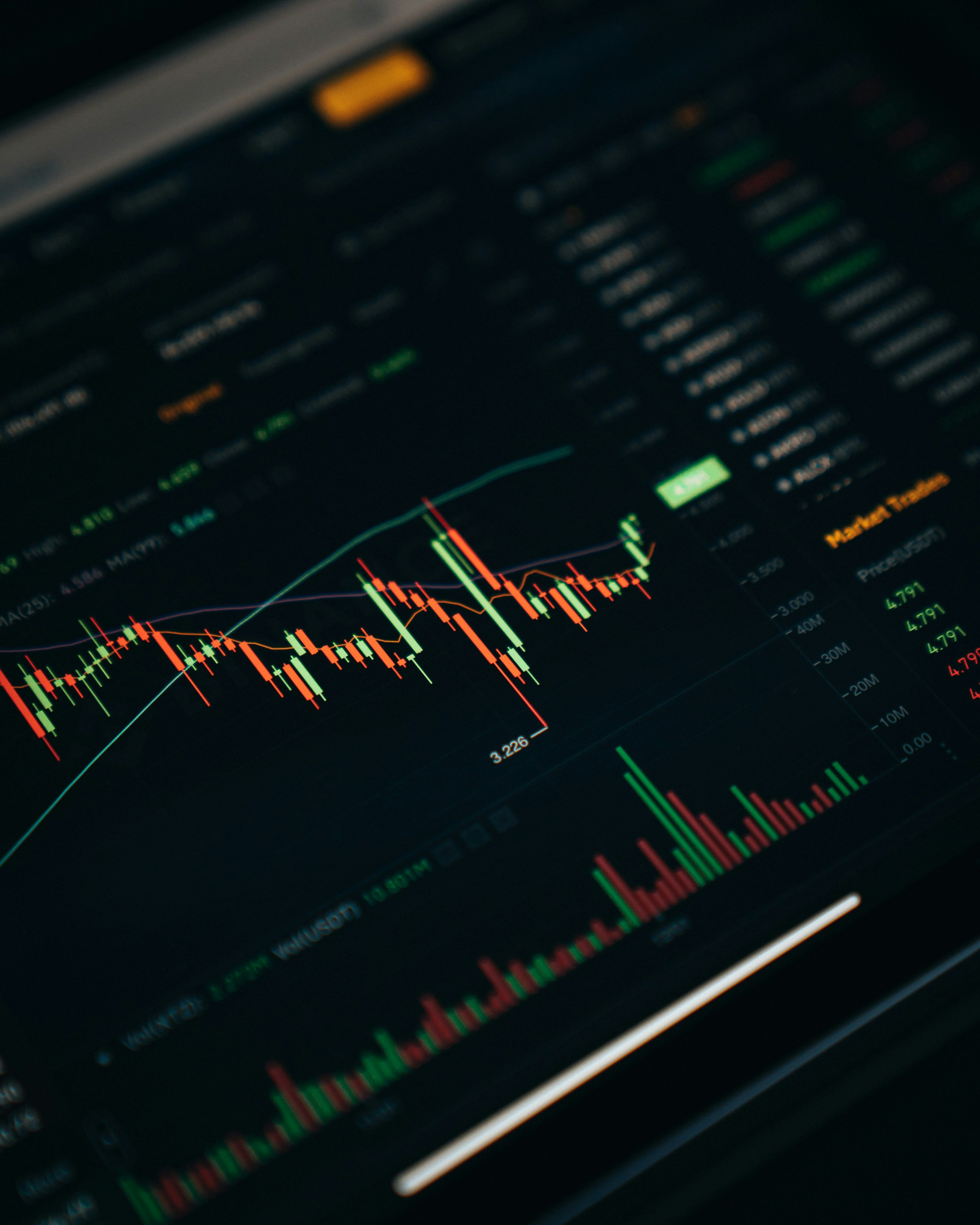# How to Use IV Value to Trade Options Like a Pro

IV value, or implied volatility, is a measure of how much volatility the market is expecting in the underlying asset. It is calculated using a variety of factors, including the current price of the asset, the strike price of the option, and the time to expiration. The IV value of an option can be seen by looking at the option's price. The higher the IV value, the more expensive the option will be. This is because the market is expecting more volatility in the underlying asset, and therefore the option is more likely to be in the money.

amuktha.com## How to See IV Value of an Option Price Trend

IV value, or implied volatility, is a measure of how much volatility the market is expecting in the underlying asset. It is calculated using a variety of factors, including the current price of the asset, the strike price of the option, and the time to expiration.

The IV value of an option can be seen by looking at the option's price. The higher the IV value, the more expensive the option will be. This is because the market is expecting more volatility in the underlying asset, and therefore the option is more likely to be in the money.

The IV value of an option can also be seen by looking at a volatility chart. A volatility chart shows the historical IV value of an option over time. This can be helpful for identifying trends in IV value.

Here are some of the ways to see IV value of a option price trend:

• Use a volatility chart: A volatility chart shows the historical IV value of an option over time. This can be helpful for identifying trends in IV value.

• Use an options pricing calculator: An options pricing calculator can be used to calculate the IV value of an option. This is a more accurate way to see IV value, but it can be more difficult to use.

• Look at the option's bid and ask prices: The bid and ask prices of an option are typically based on the IV value of the option. The higher the IV value, the more expensive the option will be.

It is important to note that IV value is not always a reliable indicator of future volatility. The market can change its expectations at any time, and this can lead to changes in IV value. However, IV value can be a useful tool for identifying trends in volatility and for making informed decisions about option trading.

Here are some additional tips for using IV value to trade options:

• Consider the underlying asset: The IV value of an option is based on the volatility of the underlying asset. If the underlying asset is volatile, the IV value of the option will be higher.

• Consider the time to expiration: The IV value of an option decreases as the time to expiration decreases. This is because the option has less time to become in the money.

• Consider the strike price: The IV value of an option is higher for options with strikes that are closer to the current price of the underlying asset. This is because these options are more likely to become in the money.

By following these tips, you can use IV value to make informed decisions about option trading.